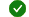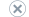# Code metrics - Maintainability index range and meaning

Applies to:Visual StudioVisual Studio for MacVisual Studio Code

Question: The maintainability index has been reset to lie between 0 and 100. How and why was this done?

The metric originally was calculated as follows: `Maintainability Index = 171 - 5.2 * ln(Halstead Volume) - 0.23 * (Cyclomatic Complexity) - 16.2 * ln(Lines of Code)`

The use of this formula meant that it ranged from 171 to an unbounded negative number. As code tended toward 0, it was clearly hard to maintain code and the difference between code at 0 and some negative value was not useful. As a result of the decreasing usefulness of the negative numbers and a desire to keep the metric as clear as possible, we decided to treat all 0 or less indexes as 0 and then rebase the 171 or less range to be from 0 to 100. For this reason, the formula we use is:

`Maintainability Index = MAX(0,(171 - 5.2 * ln(Halstead Volume) - 0.23 * (Cyclomatic Complexity) - 16.2 * ln(Lines of Code))*100 / 171)`

In addition to that, we decided to be conservative with the thresholds. The desire was that if the index showed red then we would be saying with a high degree of confidence that there was an issue with the code. This gave us the following thresholds:

For the thresholds, we decided to break down this 0-100 range 80-20 to keep the noise level low and we only flagged code that was suspicious. We've used the following thresholds:

• 0-9 = Red
• 10-19 = Yellow
• 20-100 = Green